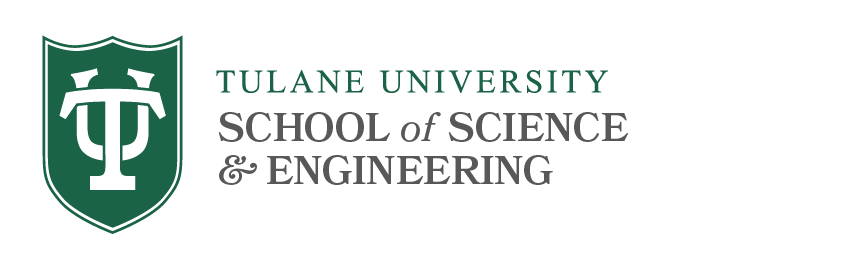# 1000 Level Courses

Math 1110 Probability & Statistics (3)
Prerequisite: High school algebra.
Elementary probability theory with applications; random variables; distributions including a thorough discussion of the binomial, Poisson, and normal distributions; central limit theorem; histograms; sampling distributions; confidence intervals; tests of hypotheses; linear models; regression.

Math 1140 Statistics for Business (4)
Prerequisite: High school algebra.
An introductory statistics course for pre-BSM students, which emphasizes basic topics on statistical inferences with extensive coverage of data collection and analysis as needed to evaluate the reported statistical results and to make good decisions in business. The course stresses the development of statistical thinking, the assessment of credibility and the value of the inferences made from data, both by those who analyze and those who produce them. In comparison with Math 1110, this course spends more time on statistical inference problems, less on probability, and teaches the use of Excel, a computer skill necessary nowadays in the business world.

Math 1150, 1160 Long Calculus (3, 3)
The material of Calculus 1210 is covered in two semesters, with diversions for topics in algebra, trigonometry, complex numbers as the need for these topics arises. Mathematics 1150 is a prerequisite for 1160. Students finishing the course sequence 1150-1160 may continue with 1220 or any other course having Calculus 1210 as a prerequisite. The combination of 1150 and 1160 may count as one course toward the B.S. degree requirement.

Math 1210 Calculus I (4)
Prerequisites: High school algebra, geometry, and trigonometry.
Functions and their graphs, limits and continuity, derivatives and applications of derivatives, and introduction to the integral.

Math 1220 Calculus II (4)
Prerequisite: Grade of at least C- in Math 1160 or 1210.
Integration; exponential, logarithmic, and trigonometric functions; techniques of integration; mean value theorem; Taylor’s Theorem and Taylor series; and infinite series.

Math 1230 Statistics for Scientists (4)
Prerequisite: Math 1210 or permission of instructor.
The objective of this course is to provide a practical overview of the statistical methods and models most likely to be encountered by scientists in practical research applications. Students will learn statistical concepts by generating and analyzing stochastic datasets using the Minitab software package. Specific topics that will be covered in this course include discrete and continuous distributions, sampling methods and descriptive statistics, the Central Limit Theorem and its applications, estimation methods, confidence intervals, hypothesis testing, linear regression, and Analysis of Variance.

Math 1310 Consolidated Calculus (4)
Prerequisite: A score of 3 or higher on the AB or BC Calculus AP test or permission of the Mathematics department undergraduate coordinator.
A combined course in Calculus I and II for students with a background in Calculus I. Students receive credit for both this course and 1210 if they receive a B- or higher. Students may not receive credit for both 1310 and 1220.